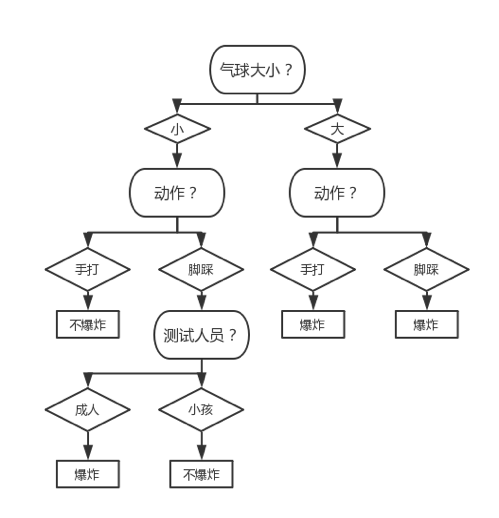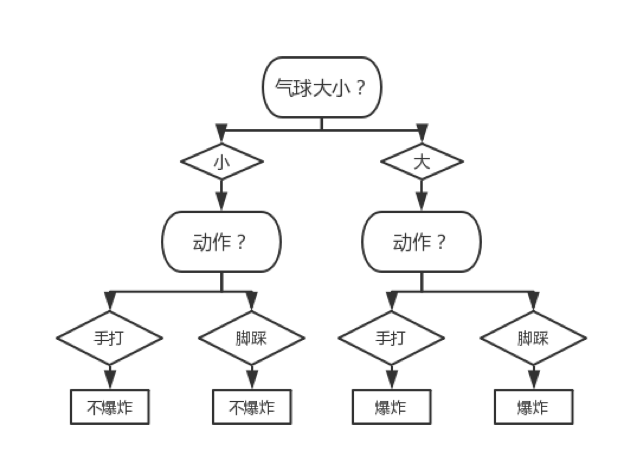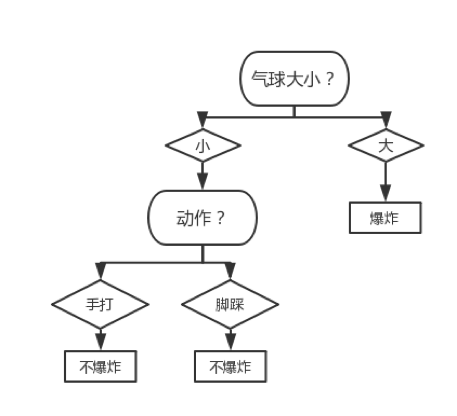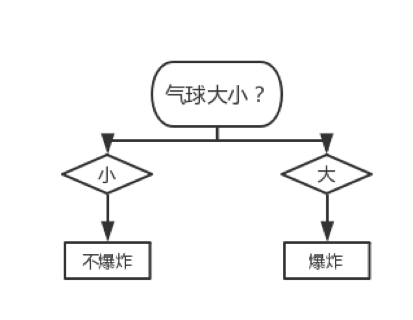• 应用交叉验证的思想，若局部剪枝能够使得模型在测试集上的错误率降低、则进行局部剪枝（预剪枝中也应用了类似的思想）
• 应用正则化的思想、综合考虑不确定性和模型复杂度来定出一个新的损失（此前我们的损失只考虑了不确定性），用该损失作为一个 Node 是否进行局部剪枝的标准

• 定义新损失（$T$代表一个 Node） 其中，$C\left( T \right)$即是该 Node 和不确定性相关的损失、$|T|$则是该 Node 下属叶节点的个数。不妨设第 t 个叶节点含有$N_{t}$个样本且这$N_{t}$个样本的不确定性为$H_{t}(T)$，那么新损失一般可以直接定义为加权不确定性：

• 直接比较一个 Node 局部剪枝前的损失$C_{\alpha}(T)$和局部剪枝后的损失$C_{\alpha}(t)$的大小，若：
• 获取一系列的剪枝阈值：$0 = \alpha_{0} < \alpha_{1} < \ldots < \alpha_{p} < + \infty$，在每个剪枝阈值$\alpha_{i}$上对相应的 Node 进行局部剪枝并将局部剪枝后得到的决策树$T_{i}$储存在一个列表中。在$\alpha_{p}$上我们会对根节点进行局部剪枝，此时剩下来的决策树$T_{p}$就只包含根节点这一个 Node。最后，通过交叉验证选出$T_{0},\ldots,T_{p}$中最好的决策树作为最终生成的决策树（注意其中的$T_{0}$即是没有剪过枝的原始树）

# ID3、C4.5 的剪枝算法

1. 输入：生成算法产生的原始决策树$T$，惩罚因子$\alpha$
2. 过程
1. 从下往上地获取$T$中所有 Node，存入列表_tmp_nodes
2. _tmp_nodes中的所有 Node 计算损失，存入列表_old
3. 计算_tmp_nodes中所有 Node 进行局部剪枝后的损失，存入列表_new
4. 进入循环体：
1. _new中所有损失都大于_old中对应的损失、则退出循环体
2. 否则，设 p 满足： 则对_tmp_nodes[p]进行局部剪枝
3. 在完成局部剪枝后，更新_old_new_tmp_nodes等变量。具体而言，我们无需重新计算它们、只需更新“被影响到的” Node 所对应的位置的值即可
5. 最后调用self.reduce_nodes方法、将被剪掉的 Node 从nodes中除去
3. 输出：修剪过后的决策树$T_{\alpha}$

• 局部剪枝前、该 Node 的损失为：
• 局部剪枝后、该 Node 的损失为： 其中• 局部剪枝前、该 Node 的损失为：

其中

• 局部剪枝后、该 Node 的损失为： 其中

$\alpha = 2$代入、知：# CART 剪枝

1. 输入：在训练集上调用生成算法所产生的原始决策树$T$，交叉验证集
2. 过程：
1. 从下往上地获取$T$中所有 Node，存入列表_tmp_nodes
2. _tmp_nodes中的所有 Node 计算阈值，存入列表_thresholds；其中，第 t 个 Node 的阈值$\alpha_{t}$应满足： 其中$C(t)$即是第 t 个 Node 自身数据的不确定性；换言之，$C_{\alpha_{t}}(T_{t})$代表着第 t 个 Node 进行局部剪枝前的新损失、$C_{\alpha_{t}}(t)$代表着局部剪枝后的新损失。由上式可求出： 此即阈值的计算公式
3. 进入循环体：
1. 将当前决策树存入列表self.roots
2. 若当前决策树中只剩根节点、则退出循环体
3. 否则，取 p 满足： 然后对_tmp_nodes[p]进行局部剪枝
4. 在完成局部剪枝后，更新_thresholds_tmp_nodes等变量。具体而言，我们无需重新计算它们、只需更新“被影响到的” Node 所对应的位置的值即可
4. 然后调用self.reduce_nodes方法、将被剪掉的 Node 从nodes中除去
5. 最后利用交叉验证、从self.roots中选出表现最好的决策树$T_{p^{*}}$
3. 输出：修剪过后的决策树$T_{p^{*}}$